SLVSE57C June   2017  – April 2018

PRODUCTION DATA.

1. Features
2. Applications
3. Description
1.     Device Images
4. Revision History
5. Device Comparison Table
6. Pin Configuration and Functions
7. Specifications
8. Detailed Description
1. 8.1 Overview
2. 8.2 Functional Block Diagram
3. 8.3 Feature Description
4. 8.4 Device Functional Modes
9. Application and Implementation
1. 9.1 Application Information
2. 9.2 Typical Application
10. 10Power Supply Recommendations
11. 11Layout
12. 12Device and Documentation Support
13. 13Mechanical, Packaging, and Orderable Information

• DSG|8
• DSG|8

#### 9.2.2.3.2 Case 2: Start-Up With Load. Output Capacitance COUT and Load Draw Current

When the load draws current during the turnon sequence, there is additional power dissipated. Considering a resistive load during start-up RL(SU), load current ramps up proportionally with increase in output voltage during TdVdT time. Equation 10 to Equation 13 show the average power dissipation in the internal FET during charging time due to resistive load.

Equation 10.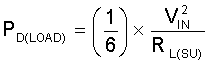Total power dissipated in the device during start-up is Equation 11.

Equation 11.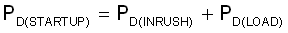Total current during start-up is given by Equation 12.

Equation 12.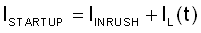If ISTARTUP> ILIMIT, the device limits the current to ILIMIT and the current-limited charging time is determined by Equation 13.

Equation 13.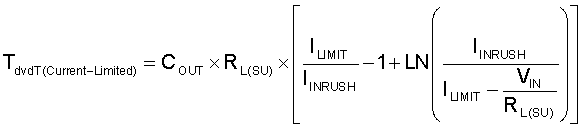The power dissipation, with and without load, for selected start-up time must not exceed the shutdown limits as shown in Figure 59.Figure 59. Thermal Shutdown Limit Plot

For the design example under discussion, select ramp-up capacitor CdVdt = OPEN. The default slew rate for CdVdt = OPEN is 38.2 mV/µs. With slew rate of 38.2 mV/µs, the ramp-up time TdVdt for 12 V input is 248 µs.

The inrush current drawn by the load capacitance COUT during ramp-up using Equation 14.

Equation 14.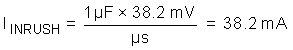The inrush power dissipation is calculated using Equation 15.

Equation 15.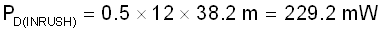For 229.2 mW of power loss, the thermal shutdown time of the device must not be less than the ramp-up time TdVdt to avoid the false trip at the maximum operating temperature. Figure 59 shows the thermal shutdown limit at TA = 85°C, for 229.2 mW of power, the shutdown time is infinite. Therefore, it is safe to use 248 µs as the start-up time without any load on the output.

The additional power dissipation when a 4 Ω load is present during start-up is calculated using Equation 10.

Equation 16.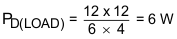The total device power dissipation during start-up is given in Equation 17.

Equation 17.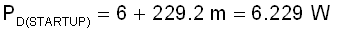The Figure 59 shows TA = 85°C and the thermal shutdown time for 6.229 W is more than 10 ms, which is well within the acceptable limits to not use an external capacitor CdVdt with a start-up load of 4 Ω.

When COUT is large, there is a need to decrease the power dissipation during start-up. This can be done by increasing the value of the CdVdt capacitor.